# How Does Data Visualization Work With Python Using Matplotlib?Data visualization plays a key role in any data science project. Businesses and organizations worldwide collect vast amounts of data almost every day and use it to make essential business decisions. But not every decision-maker has the technical proficiency to understand data in its raw form. This is where data visualization comes in. Today, data visualization using Matplotlib, a prominent library for Python, is one of the most popular avenues that analysts and data scientists take.

In this article, we will explore how to work with the different visualizations available in Matplotlib.

## What is Matplotlib?

Matplotlib was created by John Hunter during his post-doctoral research in neurobiology and released in 2003. It is the go-to Python library for graphs and visualizations. It offers a variety of plots like Line, Scatter, Bar, Histogram, Box, etc. It also supports 2D and 3D plotting.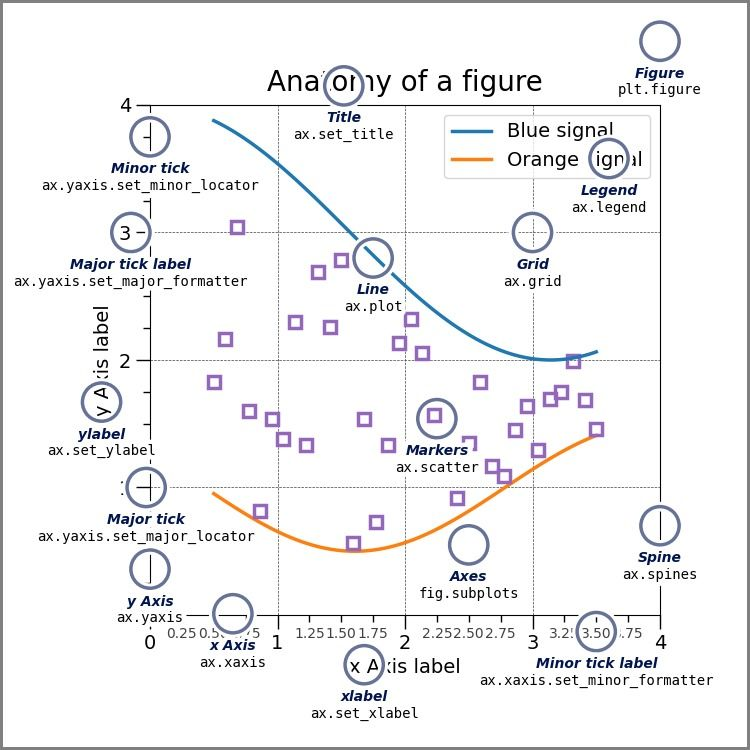Image source: Matplotlib

## Data visualization using Matplotlib

Matplotlib can installed directly from Jupyter Notebook by running the command:

``````!pip install matplotlib

``````

Or, by running this command in cmd:

``````conda install -c conda-forge matplotlib

``````

### Importing the necessary libraries

``````
import numpy as np
import pandas as pd
import matplotlib.pyplot as plt
%matplotlib inline

``````

The %matplotlib inline command is used to embed static .png images of the plot directly into the IPython Notebook.

## Customizing plots using Matplotlib

### Line styles

Let’s plot a simple line graph using sample data. We can do so using plt.plot():

``````
x = [2,4,7,8,10,15,19,22]
plt.plot(x);

``````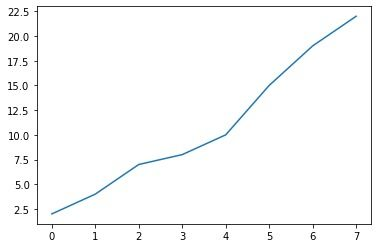Matplotlib offers a variety of linestyles that can be customized using the ls or linestyle argument in the plot(). Here’s a list of all the available options:

``````import matplotlib
matplotlib.lines.lineStyles

``````

output:

``````{'-': '_draw_solid',
'--': '_draw_dashed',
'-.': '_draw_dash_dot',
':': '_draw_dotted',
'None': '_draw_nothing',
' ': '_draw_nothing',
'': '_draw_nothing'}

``````

Let’s try out a few linestyles and some other arguments:

``````plt.plot(x,linestyle=':',color='red');

``````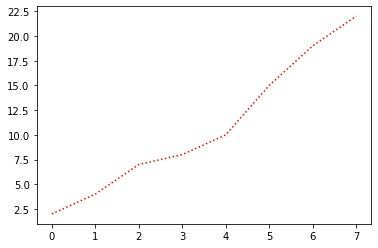Linewidth is used to change the thickness of the plot:

``````plt.plot(x,linestyle='dashdot',color='green',linewidth=5)

``````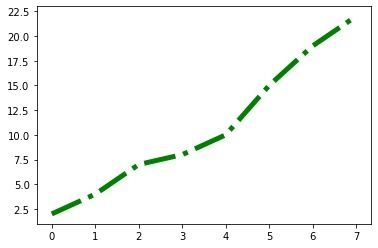### Markers

Markers are used to highlight points on the graph. Like linestyle, there’s a long list of selections of linemarkers. Here’s how they can be viewed, along with a few examples:

``````matplotlib.lines.lineMarkers
{'.': 'point',
',': 'pixel',
'o': 'circle',
'v': 'triangle_down',
'^': 'triangle_up',
'<': 'triangle_left',
'>': 'triangle_right',
'1': 'tri_down',
'2': 'tri_up'}

plt.plot(x, marker='X');

``````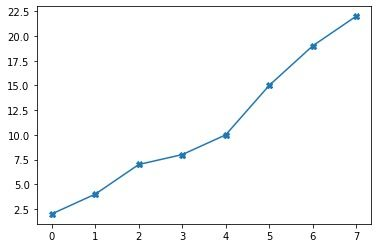We can change the size of the markers using the argument markersize.

``````plt.plot(x, marker='|',markersize=15);

``````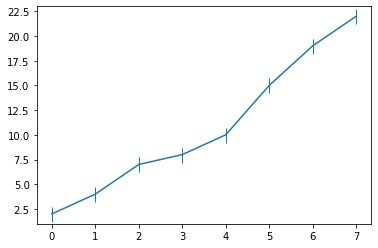Markerfacecolor is used to change the color of the marker to highlight it more, and markeredgecolor is used to change the borders:

``````plt.plot(x, marker='o', markersize=10, markeredgecolor='black',
markerfacecolor='yellow');

``````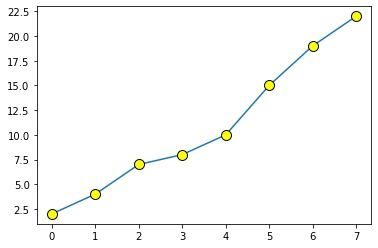### Title and axes labels

Most times, it’s necessary to add texts or labels to the axes of the graphs to help viewers understand what the plot is actually about. In Matplotlib, we do this using xlabel() and ylabel().

Here’s our sample data to show the monthly sales of a company:

``````
months = ['Jan', 'Feb', 'March', 'Apr', 'May', 'June']
sales = [10789, 12897, 13554, 12650, 14320, 15236]

plt.plot(months,sales,linewidth=3)
plt.xlabel('Month')
plt.ylabel('Sales')
plt.title('Monthly Sales');

``````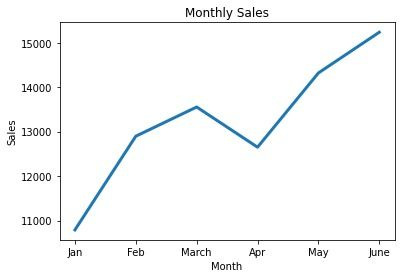### Legend

When plotting multiple lines in a graph, legends are used to describe the different elements using matplotlib.pyplot.legend().

Loc is used to specify the location of the legend index. The values can be ‘upper left’, ‘upper right’, ‘lower left’, and ‘lower right’ of the corresponding graph.

``````salesA = [10789, 12897, 13554, 13650, 14320, 15236]
salesB = [10364, 13326, 14569, 14987, 15005, 15241]
salesC = [9891, 11369, 11659, 12458, 12430, 13985]

plt.plot(months,salesA,linewidth=2)
plt.plot(months,salesB,linewidth=2)
plt.plot(months,salesC,linewidth=2)

plt.xlabel('Month')
plt.ylabel('Sales')
plt.title('Monthly Sales Comparison')
plt.legend(labels=['Company A', 'Company B', 'Company C'], loc='upper left');

``````### Grid

The plt.grid() function is used to add a grid to the plots. Let’s add one to the Monthly Sales Comparison Plot:

``````plt.plot(months,salesA,linewidth=2)
plt.plot(months,salesB,linewidth=2)
plt.plot(months,salesC,linewidth=2)

plt.xlabel('Month')
plt.ylabel('Sales')
plt.title('Monthly Sales Comparison')
plt.legend(labels=['Company A', 'Company B', 'Company C'], loc='upper left')
plt.grid(True);

``````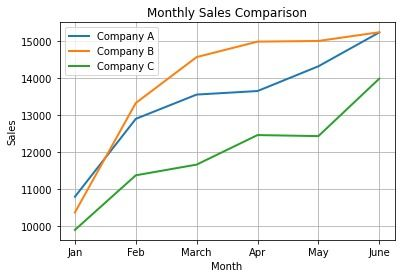This is the default grid that gets added if we don’t use any customization. A few common attributes we can use are:

• Color: To change the color of the grid lines.

• Alpha: To change the visibility of the grid lines.

• Linewidth: To alter the thickness of the grid lines.

• Linestyle: To change the line style of the grid lines.

``````plt.plot(months,salesA,linewidth=2,marker='o')
plt.plot(months,salesB,linewidth=2,marker='o')
plt.plot(months,salesC,linewidth=2,marker='o')

plt.xlabel('Month')
plt.ylabel('Sales')
plt.title('Monthly Sales Comparison')
plt.legend(labels=['Company A', 'Company B', 'Company C'], loc='upper left')
plt.grid(color='red', alpha=0.2, linewidth=2);

``````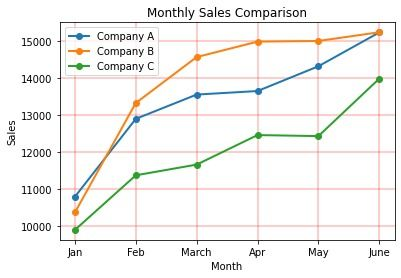### Plot dimensions

We can change the dimensions of the graph using the figsize argument in plt.figure().

``````x = np.linspace(0, 2, 200)

fig = plt.figure(figsize=(12, 6))

plt.plot(x, x, label='linear',linewidth=3)
plt.plot(x, x**3, label='cubic',linewidth=3)

plt.grid(True)
plt.title("Simple Plot")
plt.legend();

``````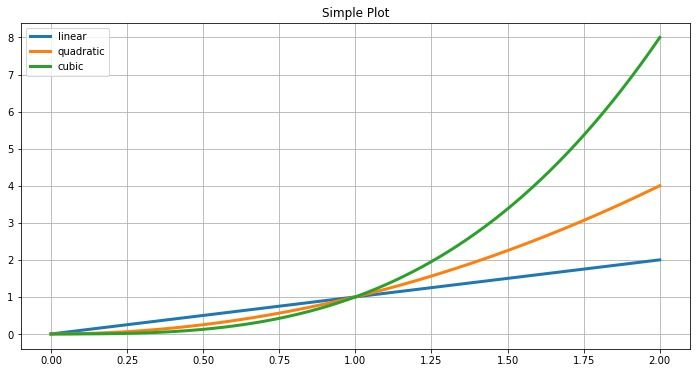Now that we’ve learned the basics of customization using line graphs, we will now cover the other different types of plots and graphs that assist with data visualization.

We will use a dataset to simulate data visualization of a real-life project. For this article, we will use the Titanic Dataset.

``````df = pd.read_csv('../input/titanic/train.csv')

``````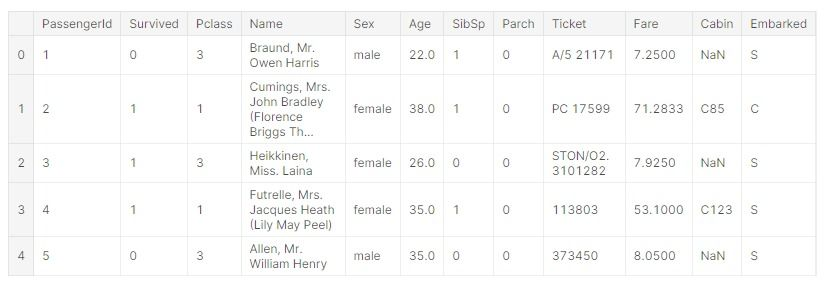Before beginning data visualization work with Python using Matplotlib, let’s get familiar with all the columns of the dataset:

• PassengerId: A unique number assigned to each passenger

• Survived: Whether the passenger survived the sinking (0 = No, 1 = Yes)

• Pclass: Passengers’ cabin class that can be used as an indicator of their socioeconomic background. (1 = first, 2 = second, 3 = third)

• Name: Name of the passenger

• Sex: Gender of the passenger

• Age: The passenger’s age

• SibSp: # of siblings or spouses aboard

• Parch: # of parents or children aboard

• Ticket: Ticket number of the passenger

• Fare: Ticket fare

• Cabin: Cabin number of passengers

• Embarked: Port passengers embarked from (S = Southampton, C = Cherbourg, Q = Queenstown).

## Types of plots

### Scatter plot

In a scatter plot, the data points are represented individually using dots or circles. This type of plot is generally used to represent the relationship between two variables.

Function used - plt.scatter()

``````age_m = df[df['Sex'] == 'male']['Age']
fare_m = df[df['Sex'] == 'male']['Fare']
age_f = df[df['Sex'] == 'female']['Age']
fare_f = df[df['Sex'] == 'female']['Fare']

fig = plt.figure(figsize=(12, 6))
plt.scatter(x=age_m, y=fare_m,s=25)
plt.scatter(x=age_f, y=fare_f,s=25)
plt.legend(['male', 'female']);

``````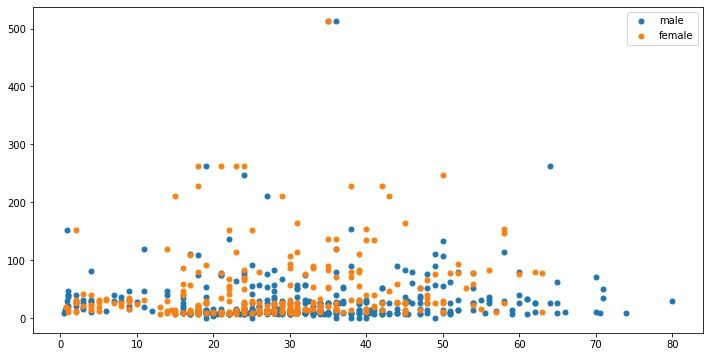We can also represent a third axis using different shades for the data points. This can be achieved using cmap.

``````pclass = df[df['Sex']=='male']['Pclass']

fig = plt.figure(figsize=(12, 6))
plt.scatter(x=age_m, y=fare_m,s=25, c=pclass, cmap='cool')
plt.colorbar(ticks=[1, 2, 3])

plt.xlabel('Age - Male')
plt.ylabel('Fare');

``````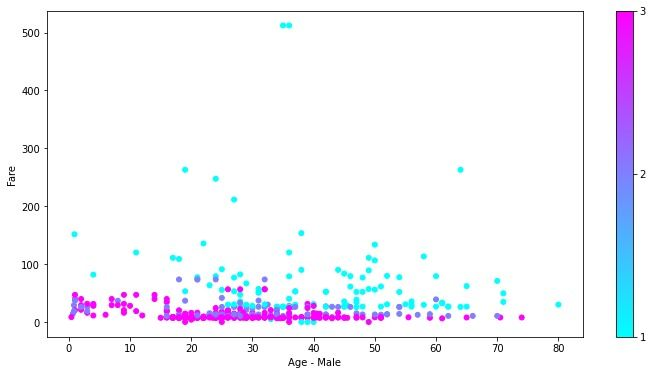### Histogram

A histogram is a bar graph-like plot used to represent the frequency distribution of a single variable. The whole range of frequencies is divided into equal units called ‘bins’.

Function used - plt.hist()

``````age = df['Age'] #distribution of passenger’s age
plt.hist(age);

``````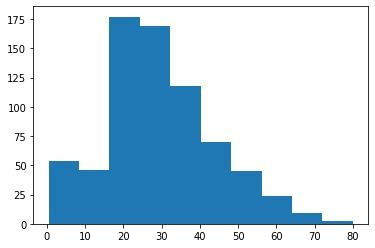We can divide the distribution into any number of bins by using:

``````plt.hist(age, bins=20)

plt.xlabel('Age')
plt.ylabel('No. of Passengers')
plt.title('Distribution of Passenger Ages')
plt.grid(True);

``````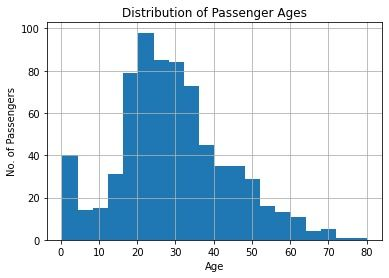### Bar graph

A bar graph is used to compare categorical data, where the height of the bars represents its value.

Function used - plt.bar()

Let's see how many people embarked from each port:

``````emb = df['Embarked'].value_counts()

plt.bar(x=emb.index, height=emb.values, width=0.5);

``````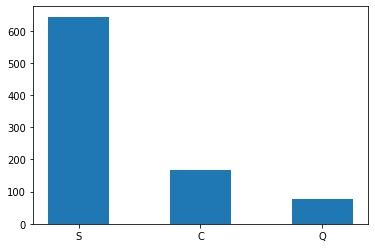We can customize the bars using additional arguments.

Let’s compare the number of people who survived and those who did not:

``````survivor = df['Survived'].value_counts()

plt.bar(x=[0,1], height=survivor.values, width=0.2, color='yellow', edgecolor='red',linewidth=2);
print('Thus the majority didn’t survive.')

``````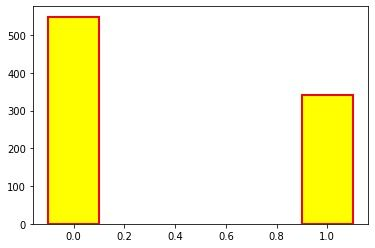Thus the majority did not survive.

#### Horizontal bar graph

We can plot bar graphs horizontally too.

Let’s compare the male and female survivors using two subplots:

``````m_survivors = df[df['Sex']=='male']['Survived'].value_counts()
f_survivors = df[df['Sex']=='female']['Survived'].value_counts()

fig, axes = plt.subplots(1, 2) #for subplots
fig.set_size_inches(8,4)

axes.barh(y=m_survivors.index, width=m_survivors.values, height = 0.2, color=['orange', 'blue'])
axes.set_title('Male Survivors')

axes.barh(y=f_survivors.index, width=f_survivors.values, height = 0.2, color=['blue', 'orange'])
axes.set_title('Female Survivors');

``````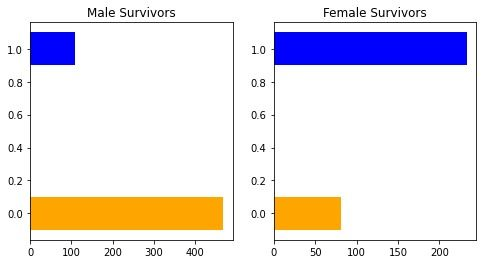#### Stacked bar graph

A stacked bar graph further divides the variable we are comparing into smaller parts to represent the different categories of the variable.

Let’s compare the ratio of men and woman who boarded the ship from different ports:

``````embarked_m = df[df['Sex']=='male']['Embarked'].value_counts()
embarked_f = df[df['Sex']=='female']['Embarked'].value_counts()

plt.bar(x=embarked_m.index, height=embarked_m.values)
plt.bar(x=embarked_f.index, height=embarked_f.values, bottom=embarked_m.values, color='orange')

plt.xlabel('Port')
plt.ylabel('Passengers')
plt.title('Embarking Ports by Sex')
plt.legend(labels=['Male', 'Female']);

``````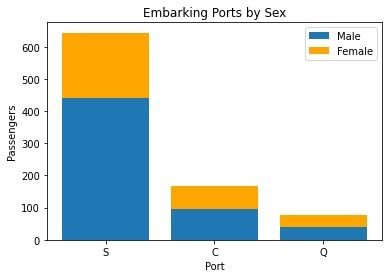### Pie chart

A pie chart is a circle divided into slices, where each slice represents the different categories under that variable. The size of the slices depends on the relative percentage or count of the categories.

Function used - plt.pie()

Let’s compare the number of men and women present onboard:

``````plt.pie(df['Sex'].value_counts(),labels=['Male','Female']);

``````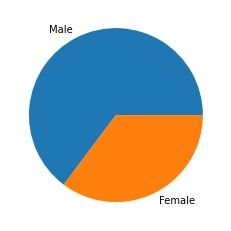We can make further specifications to make it more readable:

``````plt.pie(df['Sex'].value_counts(),labels=['Male','Female'], startangle=90,
autopct='%.1f%%', explode=[0.1, 0]);

``````### Box plot

A box plot is used to display a summary of the distribution, such as maximum, minimum, first quartile, median, third quartile, and outliers, if present.

Here’s the anatomy of a box plot: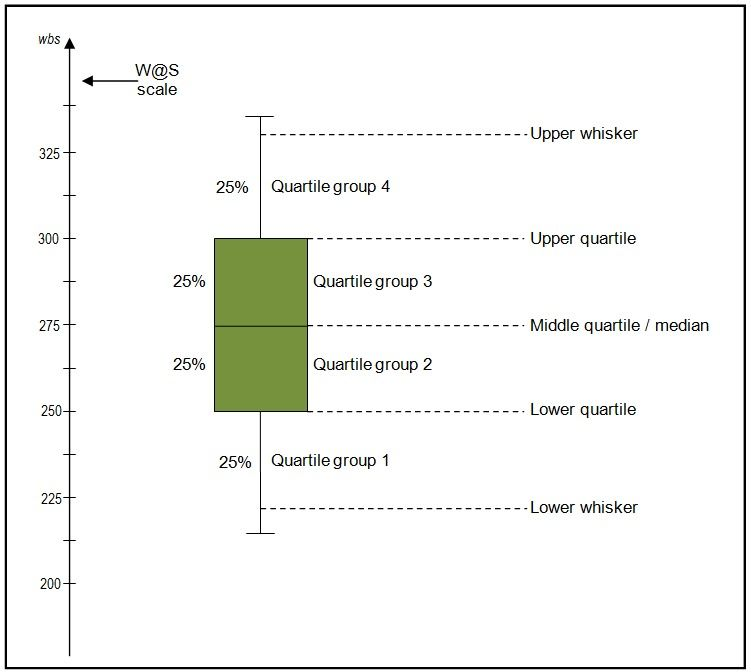Image source

Function used: plt.boxplot()

``````age = df['Age'].dropna()

fig = plt.figure(figsize=(8, 6))
plt.boxplot(age, labels=['Age']);

``````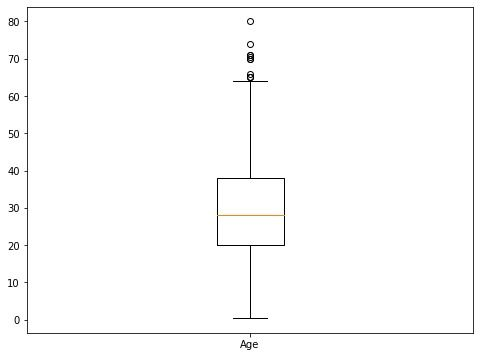From the above box plot, we can infer that the median age of the passengers was around 28; the oldest person onboard was around 80; and the youngest wasn't even a year old.

### Area chart

An area chart is essentially a line graph with the area under the curve colored or shaded. It is used to represent changes in quantity over time.

Function used - plt.fill_between()

``````x = range(20)
y = [i**2 for i in x]

plt.figure(figsize=(8,6))
plt.fill_between(x,y);

``````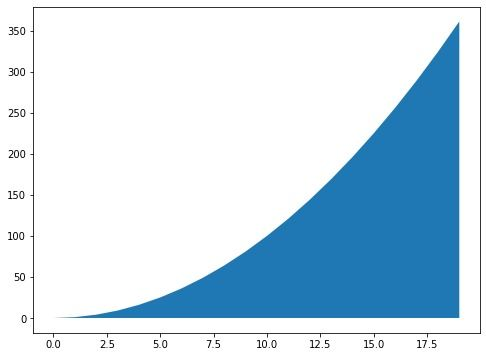### Contour plot

A contour plot is used to plot 3D surfaces on a 2D plane using color intensities to represent the third axis.

Function used - plt.contour(), plt.contourf()

``````M = np.random.rand(10,20)
plt.contour(M);

``````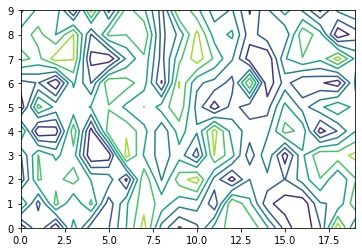To fill the contour plot:

``````plt.contourf(M);

``````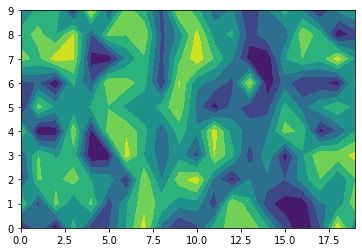### 3D plot

Three axes are used to find relationships between three variables. There’s no separate function for this, but we can add a third variable and Matplotlib will automatically plot it on a 3D plane.

Let’s make a scatter plot to find the relationship between Age Fare and Pclass variables:

``````age = df['Age']
fare = df['Fare']
pclass = df['Pclass']

fig = plt.figure(figsize=(10,8))

X = plt.axes(projection='3d')
X.scatter(xs=age, ys=fare, zs=pclass)

X.set_xlabel('Age')
X.set_ylabel('Fare')
X.set_zlabel('Class');

``````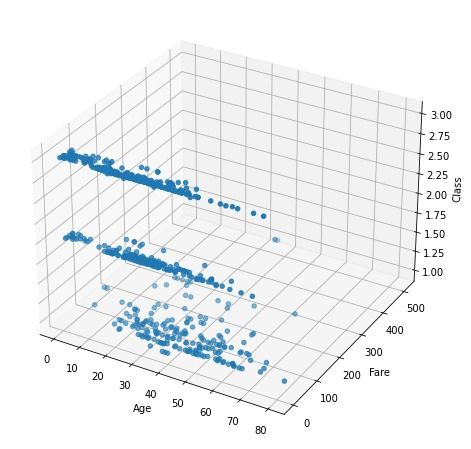## Subplots

Matplotlib offers a way to chart multiple data visualizations near each other using the plt.subplot() function.

plt.subplot(1,2) means one row with two plots.

Similarly, if we want to plot four different graphs where each row has two plots in it, we’ll use plt.subplot(2,2).

``````sex = df['Sex'].value_counts()
emb = df['Embarked'].value_counts()

fig, axes = plt.subplots(1, 2)
fig.set_size_inches(8, 4)

axes.pie(sex, labels=['Male', 'Female'], startangle=90, autopct='%.1f%%')
axes.set_title('Sex')

axes.pie(emb, labels=['S', 'C', 'Q'], startangle=90, explode=[0, 0.1, 0])
axes.set_title('Embarked');

``````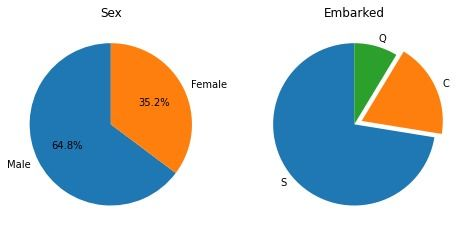We can merge different types of plots together too.

``````fig, axes = plt.subplots(1, 3)
fig.set_size_inches(12, 4)

axes.pie(s_vals, labels=['Male', 'Female'], startangle=90, autopct='%.1f%%')
axes.set_title('Sex')

age = df['Age'].dropna()
axes.boxplot(age, labels=['Age']);

age = df['Age']
fare = df['Fare']
axes.scatter(x=age, y=fare);

``````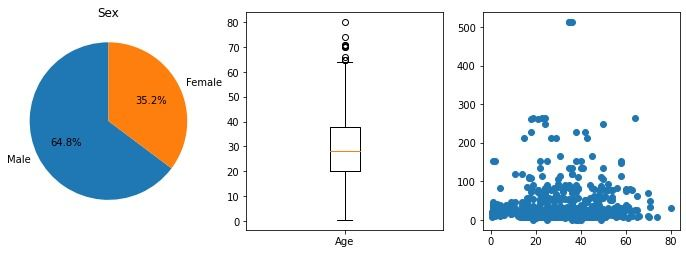## Text and annotations

Sometimes, titles, legends, and axes’ names are not enough to convey all the information we want to deliver.

We can add text directly in our plot using plt.text():

``````X = np.linspace(start=-np.pi, stop=np.pi, num=200)
Y = np.sin(2*x)

fig = plt.figure(figsize=(8, 5))
plt.plot(X,Y)
plt.text(-1.80, 0.5, 'y = sin(2x)', fontsize=14);

``````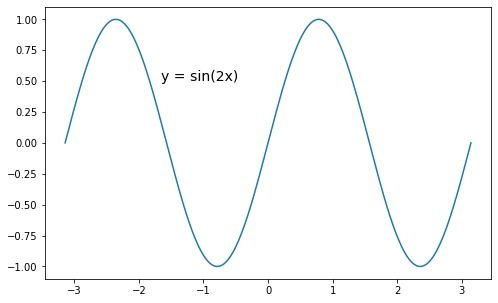We can also add arrows for more accurate annotations:

``````fig = plt.figure(figsize=(8, 5))
plt.plot(X, Y, color='gray')

plt.plot(, , 'o')

pointer = dict(facecolor='black', shrink=0.1, width=3)
plt.annotate('origin point',
xy=(0, 0),             #location of marker
xytext=(-1.80, 0.5),   #location of text
arrowprops=pointer ,   #pointer arrow
fontsize=10
);

``````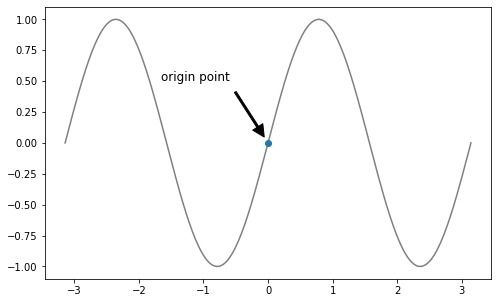We’ve explored data visualization using Matplotlib in depth and examined the important features available. Although Matplotlib has many more functionalities, the ones laid out in this article will help you get started. Try them out before moving on to more complex visualizations.

## Author

•### Turing

Author is a seasoned writer with a reputation for crafting highly engaging, well-researched, and useful content that is widely read by many of today's skilled programmers and developers.

Data visualization’s primary role is to help non-technical people consume and understand large quantities of data. When presented properly, it helps draw actionable conclusions more easily and readily.

Matplotlib is the most popular Python module (or library) to make plots and visualizations. Since it’s the first ever Python visualization library, a number of other visualization tools are built and extended from it, for example, Seaborn, Astropy, mplfinance, PyART, etc.

Not every decision-maker in an organization has the necessary technical skills to understand vast amounts of data. Data visualization, therefore, helps leaders make more informed decisions to further their organizations’ progress.

Matplotlib is a collection of different visualization functions that work like MATLAB under the hood.

Python has an extensive collection of data visualization tools with domain-specific packages like Seaborn for statistical graphics, Cartopy for geospatial data processing, DNA features viewer, WCSAxes for plotting astronomical data, etc.

Python is the go-to programming language for any type of data analysis since it’s easy to learn and fast to implement. The Python script is intuitive and easy to read which makes it the best choice for a visualization tool.### Press

What's up with Turing? Get the latest news about us here.### Blog